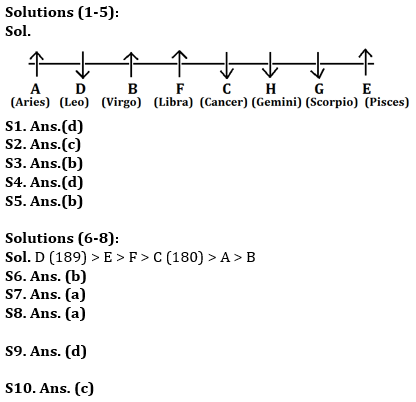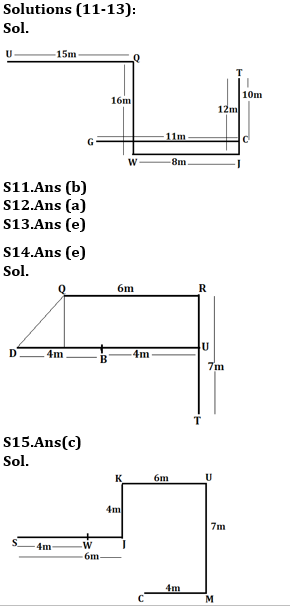Latest Banking jobs   »

# Reasoning Ability Quiz For RBI Assistant/ ESIC UDC Mains 2022- 11th April

Directions (1-5): Study the following information carefully and answer the question given below.
Eight persons are setting in a row, some of them are facing towards north direction and some of them are facing towards south direction also they like different Zodiac Sign- Pisces, Aries, Leo, Geminin, Scorpio, Virgo, Cancer and Libra (not necessarily in same order).
C sits fourth to the right of A, who is facing north direction. D sits fourth to the right of the one who likes Geminin. There are only four persons sit between E and the one who likes Virgo. G likes Scorpio sit second to the left of C. B sits second to the right of A. D and H facing same direction but opposite to E. F, who likes libra is an immediate neighbour of C and does not face south. There are only two persons sit between C and E, who is not an immediate neighbor of A. E is facing north. The one who like Aries facing north and sits fourth to the right of the one who likes cancer. G faces opposite direction to B, who faces same as F. The one who likes Pisces is an immediate neighbour of the one who likes Scorpio. H is one of the members.

Q1. Who among the following likes Pisces?
(a) D
(b) C
(c) A
(d) E
(e) None of these

Q2. Who among the following sits second to the right of the one who likes Virgo?
(a) The one who likes Aries
(b) F
(c) The one who likes Cancer
(d) G
(e) None of these

Q3. H likes which of the following Zodiac Sign?
(a) Leo
(b) Gemini
(c) Pisces
(d) Libra
(e) None of these

Q4. What is the position of F with respect to G?
(a) Fourth to the left
(b) Second to the right
(c) Immediate Left
(d) Third to the right
(e) None of these

Q5. Which of the following statement is true about H?
(a) H sits one of the extreme ends of the row
(b) H is an immediate neighbour of C
(c) B sits second to the left of H
(d) H likes Scorpio
(e) None of these

Directions (6-8): Read the following information carefully and answer the given questions.
There are six friends, each of them has different height. Height of the tallest person is 189 cm. A is taller than only B. F is shorter than only two people. C is not the tallest. E is neither taller than D nor shorter than C. The difference between the height of third shortest and the tallest person is 9. E is taller than F. F height is an odd number.

Q6. How many persons are taller than C?
(a) Four
(b) Three
(c) Two
(d) One
(e) None of these

Q7. Which of the following statement is true if height of B is 176 cm?
(a) The difference between the height of C and B is 4 cm.
(b) Height of F is 155 cm.
(c) Height of A is 154 cm.
(d) The difference between the height of D and B is 10 cm.
(e) None is true

Q8. What could be the possible height of E?
(a) 182 cm
(b) 156 cm
(c) 170 cm
(d) 198 cm
(e) 159 cm

Q9. Which of the following symbols should replace the sign (@) and (%) respectively in the given expression in order to make the expression X < U and R ≥ S definitely true?
P≥X<Q≤T@U=R≥V%S
(a) ≤, >
(b) ≤, ≤
(c) >, ≤
(d) =, ≥
(e) ≥, <

Q10. Which of the following will be definitely true if the given expression ‘A≥D>C=B≥G<E=F≤K’ is definitely true?
(a) A≥G
(b) C<E
(c) K>G
(d) D≥F
(e) B=D

Directions (11-13): Study the following information carefully and answer the questions given below.
P%Q (5)- P is 7m in south of Q
P#Q (4)- P is 6m in west of Q
P&Q (2)- P is 4m in north of Q
P@Q (8)- P is 10m in east of Q

Q@U(13), T&C(8), W%Q(14), T&J(10), J@W(6), C@G(9)

Q11. What is the direction and shortest distance of point G with respect to point U?
(a) 25m, South-west
(b) √340m, South-east
(c) 18m, South-East
(d) 16m, North
(e) None of these

Q12. What is the direction of point Q with respect to point C?
(a) North-west
(b) South-east
(c) North
(d) South
(e) None of these

Q13. If Point S is 13m West of point G then What is the direction of S with respect to U?
(a) South
(b) North
(c) North east
(d) East
(e) None of these

Directions (14-15): Each of these questions is based on the following information:
(i) A & B means A is 6m west of B.
(ii) A % B means A is 4m South of B.
(iii) A * B means A is 7m North of B.
(iv) A @ B means A is 4m East of B.

Q14. If the expression ‘Q&R*T%U@B@D’ is true, what is the Shortest distance between D and Q?
(a) 5m
(b) 7m
(c) 4m
(d) 6m
(e) None of these

Q15. If the expression ‘W@S&J%K&U*M@C’ is true, what is the direction of J with respect to M?
(a) West
(b) North
(c) North West
(d) South
(e) None of these

Solutions